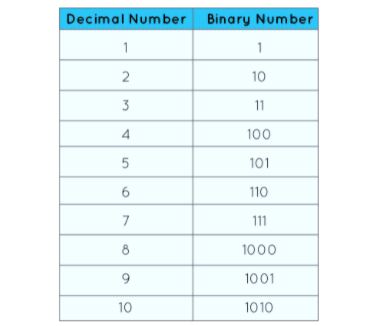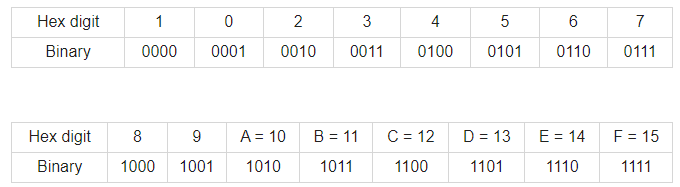Binary number system, Decimal number system, Octal number system, Hexadecimal number systems : Pharmaguideline -->
 Editable Pharmaceutical Documents in MS-Word Format

# Binary number system, Decimal number system, Octal number system, Hexadecimal number systems

In binary system, a number is defined using binary number system. The binary system uses only two digits, 0 and 1, to represent a number.

## Binary Number System

In binary system, a number is defined using binary number system. The binary system uses only two digits, 0 and 1, to represent a number. Many computer languages use binary number systems, including Java and C++. The computer can only understand binary language (0 or 1), which is 0s and 1, so all input is translated into a series of 0s and 1s before further processing. To make sense of the information in this lesson, I have converted a decimal number to a binary number and then returned the binary number to a decimal number.

The binary symbol "Bi" means "two". The line is thus drawn back to 0 and 1 as the only ways to represent a number. Binary numbers can be expressed easily in terms of decimal numbers. Differences between binary and decimal number systems. Binary numbers are represented by their base of two, while decimal numbers are represented by their base of 10.

Following is how 1 through 10 are expressed in a binary number system:## Decimal Number System

Numbers are represented by their bases in the number system. There are four bases for numbers: binary, octal, decimal, and hexadecimal. If the base is 2, it is binary, if it is 8, it is octal, if it is 10, it is decimal, and if it is 16, it is hexadecimal. Taking decimal numbers and converting them to another numbering system is easy. However, converting decimal numbers from other numbers systems requires practice.

We will examine in this article more about decimal numbers and how they can be converted to other systems in detail. Basis 10 represents decimal numbers in the decimal number system. Also known as decimal notation, it is a way of representing decimal numbers with base 10. Many computer programs utilize this number format. Base-10 is a number system consisting of 10 digits, for example, 0,1,2,3,4,5,6,7,8,9. Decimal numbers have a distinct position for each digit, and every digit is 10 times more significant than any previous digit. Two is ten times more than five when 25 is a decimal number. A decimal system number is one that represents a number from 0 to 9 using digits from 0 to 9.

In base-10 notation, the number is written with the zero or first nine positive integers representing each value. Powers of 10 are placed at the end of each value. Having ten times the size of the digit at the tens place means it is ten times as large as the tenth at the unit place. Counting in decreasing order of power each digit that follows a decimal zero (.) in a decimal number.

## Octal Number System

With the Octal Number System, 0 through 7 are arranged as eight-digit numbers. Whenever octal numbers are grouped in threes, binary numbers are used as a representation. Number systems that have a base of 'eight' are called octal numbers. This system works with zero to seven numbers. Based on the base 2, a binary number is a decimal, based on the base 10, and based on the base 16, a hexadecimal number. Base 2 is the same as base 8 when it comes to describing an octal number.

By grouping successive binary numbers into groups of three (for integers, starting from the right), octal numerals are easily converted from binary representations (similar to quaternary numerals). Binary representation of decimal 74 is 1001010, for example. By adding two zeros at the left: (00)1 001 010, which corresponds to the octal numbers 1 1 2, one gets the octal number 112.

A base 8 number, such as 248 or 1098 or 558, is an octal number. Only seven digits are used in the octal number system. Beyond 7, like 8 or 9, octal digits are not used. One divided by 10 is called an octal number. The number 19 does not represent an octal number.

In the Hexadecimal Number System, there is a value of base 16 for the Number Representation technique. The only possible values are 0, 1, 2, 3, 4, 5, 6, 7, 8, 9, A, B, C, D, E, F. For example, A is a single bit representation of decimal values 10, 11, 12, 13, 14 and 15 while B is a double bit representation of decimal values 14 and 15. Any digit's value can be represented with only 4 bits. 0x prefixes and h suffixes are used as hexadecimal notation. The weight of every digit in a power of 16.

Hexadecimal numbers have 16 times the significance of preceding positions, so to calculate the value of a hexadecimal number, multiply each digit with the value of the position it appears, and then add the products. Besides a weighted system of numbers, we also have a positional number system. There are only four bits used for hexadecimal numbers, with each group of bits having a different value between 0000 (for 0) and 1111 (for F = 15 = 8+4+2+1). Below is a table summarized in binary form of Hexadecimal number equivalents.Like Octal numbers, Hexadecimal numbers are also a system of numbers. By using hexadecimal numbers, it is possible to convert large binary numbers into smaller, more compact groups.
Get subject wise printable pdf documents

Ankur Choudhary is India's first professional pharmaceutical blogger, author and founder of pharmaguideline.com, a widely-read pharmaceutical blog since 2008. Sign-up for the free email updates for your daily dose of pharmaceutical tips.

## CURRENT JOBS

 Show All ❭❭Jobs by PharmaJobs

## DOCUMENTS

PHARMACEUTICAL DOCUMENTS

Editable Pharmaceutical Documents in MS-Word Format. Ready to use SOPs, Protocols, Master Plans, Manuals and more...

View